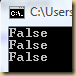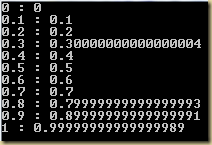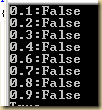## Tuesday, May 12, 2009

### Quick, what is the output of “(0.1 + 0.2) == 0.3”

So what do you think would be the output from the following lines?

`Console.WriteLine((0.1 + 0.2) == 0.3);Console.WriteLine((2.0 + 0.53) == 2.53);Console.WriteLine(1/10 == 0.1);`

They will all result in a “FALSE”!If you are not surprised, then move along there is nothing new for you to learn here.

The problem arises from the fact that doubles and floats are stored in memory using the binary system which is a base 2 system. The base 2 system cannot represent some decimal numbers, for example 0.1. This is the reason that the above logical expressions all evaluate to false, even though they look like they should evaluate to true.

Check out the output of the following code (notice the “r” in the ToString):

`for (double i = 0.0; i < 1.0; i=i+0.1)   Console.WriteLine(i + " : " + i.ToString("r"));`Each of the numbers that did not display as the original number are all numbers that cannot be represented in binary. These numbers are then stored using an approximation, which shows up when you display the numbers using the “round-trip” notation in .Net.

Why should you be cognizant of the above fact?

If you ever think that you might need to compare two double or float numbers, then you might come across cases shown above and your results might not be exactly what you expect (eg: 1/10 is not equal to 0.1).

So what should you do, when you think you might have to compare two numbers? Use the Decimal type in .Net. The Decimal type stores numbers using the base 10 system, which is the system of the numbers we are using in the first place. For this reason, Decimal types are capable of storing numbers with much higher precision.

Thus the result of:

`Console.WriteLine((0.1M+0.2M) == (0.3M));`

Will be: True (M represents Decimal type)

If you will be storing numbers that represent money or will be used to act upon currency values, then you will be better off using the Decimal type. But also be aware of the fact that the higher precision comes at a cost of speed. Computations using Decimal type values can be quite a bit slower than numbers stored using a format that is native to the computer (read binary – float and doubles).

Update (05.28.2009)
The comment below is correct. My code above demos the problem that this non represent-ability of some decimal fractions poses. Here is the complete list of numbers between 0.0 and 1.0 (incremented by 0.1) that are not represent-able in binaryUpdate 2:

Here is some code to determine if a decimal number is represent-able in binary or not:

```static bool canBeRepresentedInBase2(decimal pNumberInBase10)
{
//check if a number in base 10 can be represented exactly in base 2
//reference: http://en.wikipedia.org/wiki/Binary_numeral_system
bool funcResult = false;

int nbOfDoublings = 16*3;
decimal doubledNumber = pNumberInBase10;
for (int i = 0; i < nbOfDoublings ; i++)
{
doubledNumber = 2*doubledNumber;
decimal intPart;
decimal fracPart = ModF(doubledNumber/2, out intPart);
if (fracPart == 0) //number can be represented exactly in base 2
{
funcResult = true;
break;
}
}
return funcResult;
}

static decimal ModF(decimal number, out decimal intPart)
{
intPart = Math.Floor(number);
decimal fractional = number - (intPart);
return fractional;
}```

And you call it like this:
WL(canBeRepresentedInBase2(0.5M));
WL(canBeRepresentedInBase2(2.05M));
WL(canBeRepresentedInBase2(1.236M));
WL(canBeRepresentedInBase2(0.4M));

Reference:

What every computer scientist should know about floating point arithmetic
http://docs.sun.com/source/806-3568/ncg_goldberg.html

Floating Point in .NET: Concepts and Formats
http://www.extremeoptimization.com/resources/Articles/FPDotNetConceptsAndFormats.aspx

Binary floating point and .Net
http://www.yoda.arachsys.com/csharp/floatingpoint.html

.Net Decimal data type
http://msdn.microsoft.com/en-us/library/system.decimal.aspx